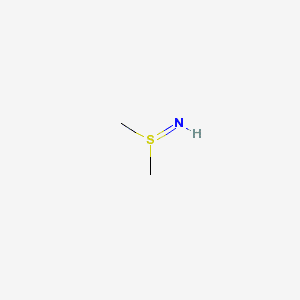## Saturday, May 03, 2008

### Wicked chemistry and unit testing

After a discussion on starting development releases for CDK on cdk-devel, the discussion continued on the state of the CDK atom typer. Dan and Rajarshi have done tests in the past against PubChem and its DTP/NCI subset. Rajarshi made his analysis part of CDK Nightly, and provides but a summary (which seems broken: zero fails) and a detailed list.

Dan, do I understand correctly that those Structure Evaluation:No Comparision - Unparameterized Atom - S. lines in the Depositor-Supplied Comments section on PubChem are based on CDK trunk? That would be a great honor! Anyways...

The amount of atom types we use to describe the chemistry we observe is overwhelming (even without charged or radical atoms). And, most atom type lists are quite limited in what they represent. However, having an explicit list allows the computer to decide if it can do reasonable calculations on a structure. Always filter your data to screen for unrecognized atom types, before heading of to, for example, QSAR calculations!

Now, many fails are because of the incomplete CDK atom type list (e.g. Au in SID:413374), or because the atom typer code has a bug (e.g. SID:403517). And these screenings against PubChem provide a nice priority list. However, others are either because the used SDF format cannot represent the chemistry (e.g. SID:420394), or the entry is a plain wrong (e.g. SID:301178). The latter two types of fails, I am annotating using del.icio.us/egonw/pubchem+check-valency for others to comment on (just tag the same page using del.ici.us, and I'll see the comments show up.

Unit testing
For the first two types of fails, basically three things need to be done:
1. add the atom type to the ontology
2. write a unit test for CDKAtomTypeMatcherTest
3. add perception code to CDKAtomTypeMatcher
Because we cannot use SMILES or file readers for writing these tests (than we can confounding of error sources), we have to hard code the chemical structure, which may be a bit cumbersome.

Unless you use the CDKSourceCodeWriter! This IChemObjectWriter creates CDK source code, staring with a IMolecule. Now, because our bug reports are derived from fails against the PubChem screening, we can simply use this BeanShell code to download a structure from PubChem and convert it to CDK source code:
#!/usr/bin/bshimport org.openscience.cdk.Molecule;import org.openscience.cdk.io.MDLV2000Reader;import org.openscience.cdk.io.CDKSourceCodeWriter;if (bsh.args.length == 0 || bsh.args == null) {  System.out.println("Syntax: pubchem2unittest.bsh [CID]\n");  System.exit(0);}String cid = bsh.args;String urlString = "http://pubchem.ncbi.nlm.nih.gov/summary/summary.cgi?disopt=SaveSDF&cid=" + cid;URL url = new URL(urlString);MDLV2000Reader reader = new MDLV2000Reader(url.openStream());Molecule mol = reader.read(new Molecule());StringWriter stringWriter = new StringWriter();CDKSourceCodeWriter writer = new CDKSourceCodeWriter(stringWriter);writer.write(mol);writer.close();System.out.print(stringWriter.toString());
For example, I am currently debugging a sulphur atom type perception problem, for which the simplest substructure looks like (sid=12279910, InChI=1/C2H7NS/c1-4(2)3/h3H,1-2H3):I can convert this PubChem entry to CDK source code with:
\$ bsh -classpath dist/jar/cdk-svn-20080221.jar tools/pubchem2unittest.bsh 12279910
Resulting in this output which I can copy/paste into my unit test:
IMolecule mol = new Molecule();IAtom a1 = mol.getBuilder().newAtom("S");a1.setPoint2d(new Point2d(2.866, 0.25));  mol.addAtom(a1);IAtom a2 = mol.getBuilder().newAtom("N");a2.setPoint2d(new Point2d(3.7321, 0.75));  mol.addAtom(a2);IAtom a3 = mol.getBuilder().newAtom("C");a3.setPoint2d(new Point2d(2.0, 0.75));  mol.addAtom(a3);IAtom a4 = mol.getBuilder().newAtom("C");a4.setPoint2d(new Point2d(2.866, -0.75));  mol.addAtom(a4);IAtom a5 = mol.getBuilder().newAtom("H");a5.setPoint2d(new Point2d(2.31, 1.2869));  mol.addAtom(a5);IAtom a6 = mol.getBuilder().newAtom("H");a6.setPoint2d(new Point2d(1.4631, 1.06));  mol.addAtom(a6);IAtom a7 = mol.getBuilder().newAtom("H");a7.setPoint2d(new Point2d(1.69, 0.2131));  mol.addAtom(a7);IAtom a8 = mol.getBuilder().newAtom("H");a8.setPoint2d(new Point2d(2.246, -0.75));  mol.addAtom(a8);IAtom a9 = mol.getBuilder().newAtom("H");a9.setPoint2d(new Point2d(2.866, -1.37));  mol.addAtom(a9);IAtom a10 = mol.getBuilder().newAtom("H");a10.setPoint2d(new Point2d(3.486, -0.75));  mol.addAtom(a10);IAtom a11 = mol.getBuilder().newAtom("H");a11.setPoint2d(new Point2d(4.269, 0.44));  mol.addAtom(a11);IBond b1 = mol.getBuilder().newBond(a1, a2, DOUBLE);mol.addBond(b1);IBond b2 = mol.getBuilder().newBond(a1, a3, SINGLE);mol.addBond(b2);IBond b3 = mol.getBuilder().newBond(a1, a4, SINGLE);mol.addBond(b3);IBond b4 = mol.getBuilder().newBond(a2, a11, SINGLE);mol.addBond(b4);IBond b5 = mol.getBuilder().newBond(a3, a5, SINGLE);mol.addBond(b5);IBond b6 = mol.getBuilder().newBond(a3, a6, SINGLE);mol.addBond(b6);IBond b7 = mol.getBuilder().newBond(a3, a7, SINGLE);mol.addBond(b7);IBond b8 = mol.getBuilder().newBond(a4, a8, SINGLE);mol.addBond(b8);IBond b9 = mol.getBuilder().newBond(a4, a9, SINGLE);mol.addBond(b9);IBond b10 = mol.getBuilder().newBond(a4, a10, SINGLE);mol.addBond(b10);

#### 1 comment:

1.he atom type QA report should have the proper numbers now